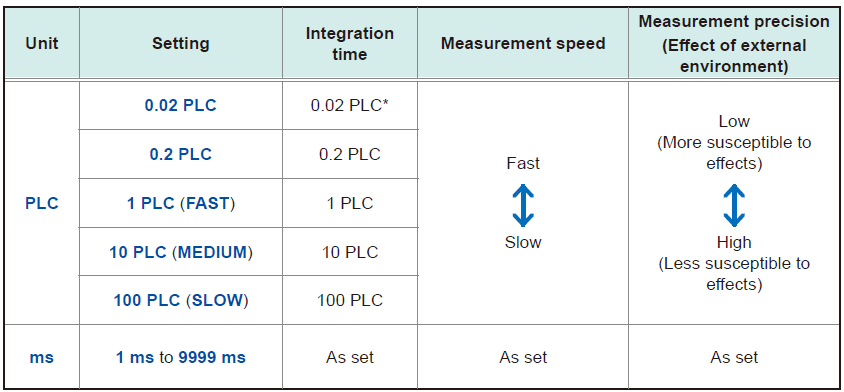# What is "1 PLC" of integration time in the measurement speed setting?

## QI'm using the Precision DC Voltmeter DM7275. What is the unit of integration time "PLC" in the measurement speed setting?

A

PLC stands for Power Line Cycle, where 1 PLC is equivalent to the duration of one cycle of the
power being supplied to the instrument. In areas with 50 Hz power, 1 PLC = 1/50 = 20 ms, while
in areas with 60 Hz power, 1 PLC = 1/60 = 16.7 ms.
In the Precision DC Voltmeter DM7275, the unit of integration time can be selected between either "ms" or "PLC".

•